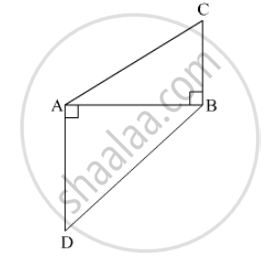# In the Given Figure, Bc ⊥ Ab, Ad ⊥ Ab, Bc = 4, Ad = 8, Then Find a ( δ a B C ) a ( δ a D B ) . - Geometry

In the given figure, BC ⊥ AB, AD ⊥ AB, BC = 4, AD = 8, then find $\frac{A\left( ∆ ABC \right)}{A\left( ∆ ADB \right)} .$#### Solution

Given:
BC = 4

$\therefore \frac{A\left( \bigtriangleup ABC \right)}{A\left( \bigtriangleup ADB \right)} = \frac{AB \times BC}{AB \times AD}$
$= \frac{BC}{AD} \left[ \because BC = 4 \text{ and } AD = 8 \right]$
$= \frac{4}{8}$

$= \frac{1}{2}$

Concept: Similar Triangles
Is there an error in this question or solution?

#### APPEARS IN

Balbharati Mathematics 2 Geometry 10th Standard SSC Maharashtra State Board
Chapter 1 Similarity
Practice Set 1.1 | Q 2 | Page 6